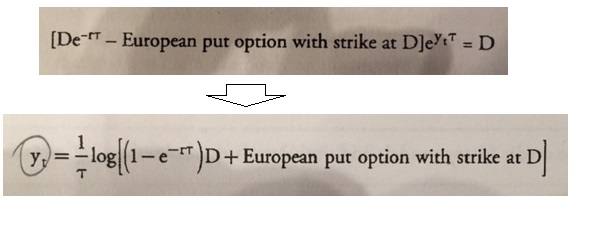What's new

# GARP.FRM.PQ.P2question in derivation process of merton model credit spread

#### nwalker

##### New Memberabove is an interim procedure from rearranging following equation:

Dt * e^yτ = D

Dt = current value of debt at time "t"
y = yield to maturity of debt
τ = remaining maturity of debt
D = debt's face value

rearranging this equation leads to the first equation in the above pic, and taking logarithm leads to the second equation in the pic (or at least is said so by the textbook). There's another procedure subtracting risk-free rate from both sides to derive credit spread, but my question concerns the migration as shown above.

As far as I understand, in order for the above process to be valid, following has to be true:
ln(1/x) = ln(1-x)

however, I cannot find any reference that this is so. Hope someone can clear this problem for me.